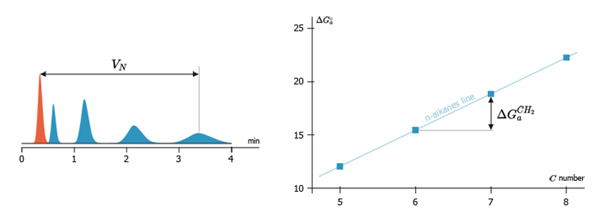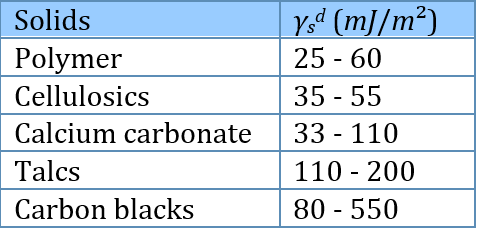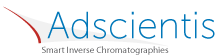## Dispersive Surface Energy

### Quick Start

The most basic surface property that can be measured via IGC is the Dispersive component. Here's how to measure it..

### Dispersive

Tmeasure °C
γsd mJ/m²A set of IGC measurements with alkane probes gives their Vg values (specific retention volumes) which in turn are normalised values calculated from retention times. From these Vg values the free energy of absorption, ΔG can be calculated via:

ΔG = RTmln(Vg)

where R is the universal gas constant, Tm is the measurement temperature (converted from °C to K).

From the slope of the curve of ΔG versus the number of carbon atoms Nc (the Dorris & Gray technique) it is possible to calculate the dispersion surface energy, γsd from:

γsd = 1/γCH2*[ΔGCH2/2N.aCH2

where N is Avogadro's number, γCH2 is the surface energy of a pure CH2 surface, given by γCH2=35.6-0.058(Τm-20), aCH2 is the area of a CH2 group (6A²) and ΔGCH2 is the (average) change in ΔG per CH2 group.

Enter your Vg values and the ΔG values and γsd are calculated for you. The data points and the least squares fit from which ΔGCH2 is calculated are also shown.

Note: just for simulation purposes the unmodified retention times can be used instead of normalized Vg. The calculated γsd will not be correct, but it can give a good impression of the behaviour of data.

Typical values of dispersive surface energies are:The IGC apps are based on the inputs kindly provided by Dr Eric Brendlé of Adscientis who are specialists in IGC measurements.